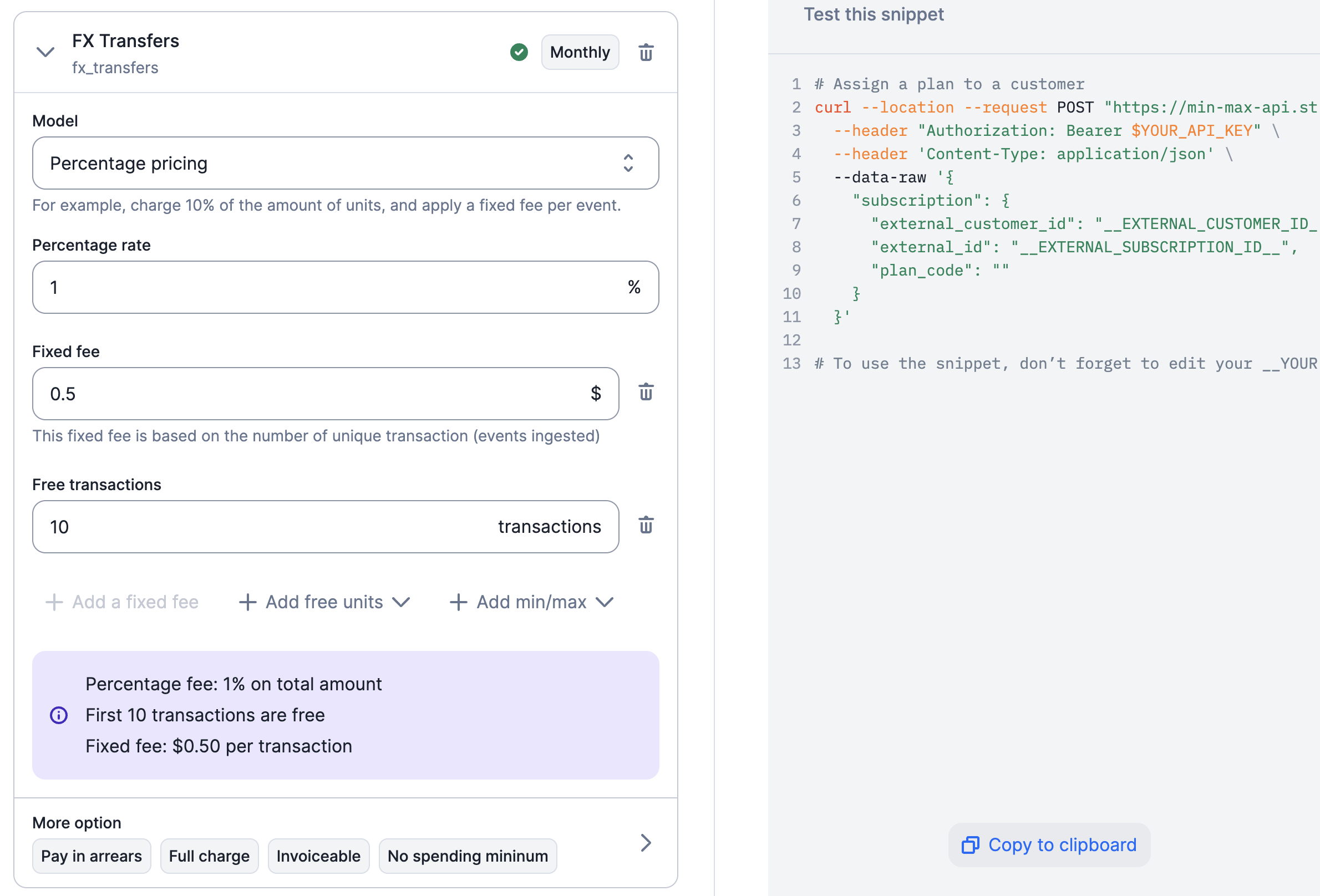Select the percentage charge model if you want to apply a percentage and a fixed fee on transactions (e.g. bank transfers, ATM withdrawals, etc.).

This charge model is generally used with billable metrics that allow users to calculate the total amount of transactions (e.g. sum aggregation type and amount defined as the event property - learn more).

You can define several parameters for the percentage charge model, including:

• Percentage rate: charge rate that applies to the total amount (e.g. 1.2% on transactions);
• Fixed fee (optional): fee that applies to each event ingested during the billing period (e.g. $0.10 per transaction); • Free units (per event - optional): number of events that are not subject to the fixed fee; • Free units (total amount - optional): amount that is not subject to the charge rate; • Per-transaction spending minimum (per event - optional): sets the minimum amount of spending required for each individual transaction; and • Per-transaction spending maximum (per event - optional): sets the maximum amount of spending required for each individual transaction. When free units are defined for both the total amount and number of events, Lago performs checks each time a new event is ingested to determine whether the free units still apply. In the illustration below for instance, the first 3 events or$500 are free.Transaction 1$2001 free event (out of 3)$200 ($500 free)No charges Transaction 2$1002 free events (out of 3)$300 ($500 free)No charges
Transaction 3$1003 free events (out of 3)$400 ($500 free)No charges Transaction 4$504 events (free units exceeded)$450 ($500 free)Charge
Therefore, for the fourth transaction, the charge will be $0.10 x 1 event + 1.2% of$50 = \$0.70.